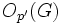# P-constrained not implies p-solvable

This article gives the statement and possibly, proof, of a non-implication relation between two group properties. That is, it states that every group satisfying the first group property (i.e., p-constrained group) need not satisfy the second group property (i.e., p-solvable group)
View a complete list of group property non-implications | View a complete list of group property implications

## Definition

It is possible to have a finite group$G$ and a prime number$p$ such that$G$ is a p-constrained group but not a p-solvable group.

## Proof

Let$G$ be the wreath product of Z2 and A5 defined as the wreath product with base group cyclic group:Z2 and acting group alternating group:A5, where we use the natural permutation action of the acting group on a set of five elements. More explicitly,$G$ is the external semidirect product of elementary abelian group:E32 and alternating group:A5 where the latter acts on the former by coordinate permutations induced by the permutations on a set of five elements.

The group$G$ has order$\! 2^5 \cdot 60 = 1920 = 2^7 \cdot 3 \cdot 5$

Let$p = 2$.

We note that:

1.$G$ is$p$-constrained: Indeed,$O_{p'}(G)$ is trivial, and$O_{p',p}(G)$ is the base of the semidirect product, i.e., a normal subgroup isomorphic to elementary abelian group:E32. In particular, this is contained in any$p$-Sylow subgroup$P$, so$P \cap O_{p',p}(G)$ is also the normal subgroup that forms the base of the semidirect product. The subgroup is a self-centralizing normal subgroup, because it is an abelian normal subgroup and the induced action by the quotient is faithful. Thus, we get the condition$C_G(P \cap O_{p',p}(G)) \le O_{p,p}(G)$.
2.$G$ is not$p$-solvable: For this, note that alternating group:A5, the simple non-abelian composition factor of$G$, is neither a$p$-group nor a$p'$-group.